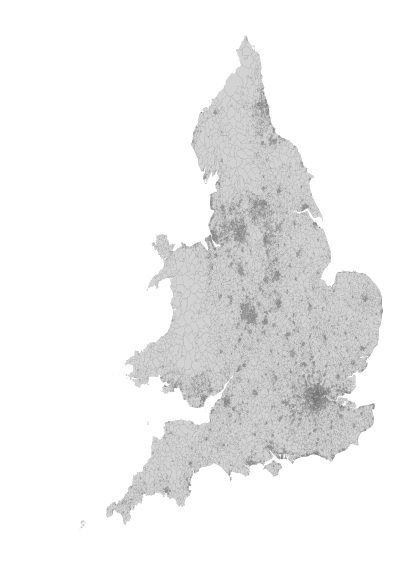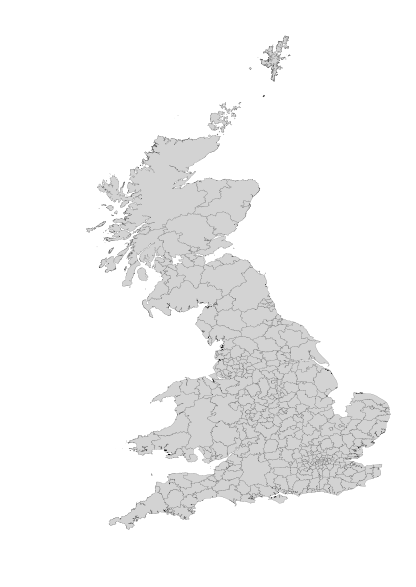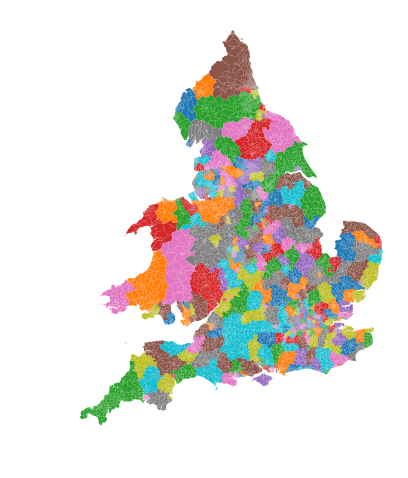In :
import pandas as pd
import numpy as np
import geopandas as gpd
import pysal as ps
import seaborn as sns
sns.set_context("talk")
import matplotlib.pyplot as plt
import sqlite3
from shapely import wkb
%matplotlib inline


# Prep & Clean the data¶

We'll use a dataset I've built for this express purpose, gathering from data sources discussed in the Appendices of the competition document.

In :
eng = sqlite3.connect('brexit_and_migrants.sql')
allofit = pd.read_sql('select * from allofit', eng)
allofit['geometry'] = allofit.the_geom.apply(lambda x: wkb.loads(x, hex=True))
allofit = gpd.GeoDataFrame(allofit)
allofit.columns = [col.replace(' - ', '') for col in allofit.columns]
allofit = allofit[allofit.lad15nm != 'Isles of Scilly'] # missing significant data here


And, we'll also read in the CDRC dataset, which I've also included in the sqlite database and joined up with LSOAs in England.

In :
lsoas = pd.read_sql('select * from lsoa_cdrc_data', eng)
lsoas['geometry'] = lsoas.the_geom.apply(lambda x: wkb.loads(x, hex=True))
lsoas = gpd.GeoDataFrame(lsoas.drop('the_geom', axis=1))


Just to show, the lsoas:

In :
f = plt.figure(figsize=(6,10))
lsoas.plot(color='lightgrey', linewidth=.05, edgecolor='k', ax=plt.gca())
plt.axis('off')
plt.show()and the local authorities:

In :
f = plt.figure(figsize=(6,10))
allofit.plot(color='lightgrey', linewidth=.1, edgecolor='k', ax=plt.gca())
plt.axis('off')
plt.show()If you look carefully, you'll see I'm missing a few local authorities. But, altogether, I've got:

In :
allofit.shape

Out:
371

of them.

### Merging the two datasets¶

Now, I'm going to merge up the data I have for the LAs with the LSOAs using geopandas, merging together the two by centroid containment in a spatial join:

In :
from geopandas.tools import sjoin

In :
joined = sjoin(allofit, lsoas.assign(geomdupe=lsoas.geometry,
centroids=lsoas.centroid)[['geometry', 'centroids', 'lsoa11cd']]\
.set_geometry('centroids').rename(columns=dict(geometry='lsoa_geometry')),
op='contains') # "trick" geopandas into taking the LSOA geometry by
# making it no longer called "geometry," and instead
# index geometries on the "centroids" column.


After the join (which you may not want to attempt if you don't have > 4 GB of memory in your computer, we can see that the assignment worked:

In :
plt.figure(figsize=(6,8))
joined.set_geometry('lsoa_geometry').plot('Area Name', ax=plt.gca())
plt.axis('off')

Out:
(105783.99201779434, 681788.2980403295, -20871.136885052583, 689838.7203237317)# Creating the Divergences¶

In this step, I'll be computing the population volatility components. First, though, I'll bring in some tools from scikit-learn and scipy, as well as a progressbar indicator to keep track of longrunning computations.

In :
import sklearn.metrics as skm
from tqdm import tqdm
from scipy import stats as st


To get a list of sorted years & ethnicities, I'm going to exploit some regularities in column structure to parse out the relevant combination of ethnicity-years in the CDRC data:

In :
years = sorted(list(set([col.split('y')[-1]
for col in lsoas.filter(regex='_y')])))
ethnicities = sorted(list(set([col.split('_')
for col in lsoas.filter(regex='_y')])))


Now, for each year, i'll

1. take that year and the next year,
2. grab all the ethnicities in both years,
3. re-normalize the distributions so they sum to one,
4. and then compute the earth-movers distance between the two vectors.
In :
for i,year in tqdm(enumerate(years[:-1])):
next_year = years[i+1]

result = np.empty(lsoas.shape)

thisyear_distribution = lsoas[[col + '_y{}'.format(year) for col in ethnicities]].copy()
isnull = np.isnan(thisyear_distribution.values).all(axis=1)
thisyear_distribution.dropna(inplace=True)

thisyear_distribution_normed = (thisyear_distribution.values) / thisyear_distribution.values.sum(axis=1)[:,None]

nextyear_distribution = lsoas[[col + '_y{}'.format(next_year) for col in ethnicities]]
nextyear_distribution_normed = (nextyear_distribution.values ) / nextyear_distribution.values.sum(axis=1)[:,None]

distances = list(map(lambda x:
st.wasserstein_distance(*x), #earthmovers distance/Wasserman distance
#                     st.energy_distance(*x), #another useful divergence
#                     st.entropy(*x) # kullback-leibler divergence
zip(thisyear_distribution_normed, nextyear_distribution_normed)))

# Here, I account for missing data distances ahead of time.
# This lets us compute the divergences only for the data we have.
result[~isnull] = distances
result[isnull] = np.nan

# if, somehow, this fails to account for all infinite divergences,
# then something is wrong with our algorithm, since the distances
# are not sane. Thus, I check explicitly.
if np.isinf(result).any():
print("divergence!")
break

# finally, I assign the volatility for y,yt+1 (named referring to the second year)
# back to the lsoa dataframe
lsoas['distmove_{}'.format(next_year)] = result


0it [00:00, ?it/s]/home/lw17329/anaconda/envs/ana/lib/python3.5/site-packages/ipykernel_launcher.py:10: RuntimeWarning: invalid value encountered in true_divide
# Remove the CWD from sys.path while we load stuff.
/home/lw17329/anaconda/envs/ana/lib/python3.5/site-packages/ipykernel_launcher.py:13: RuntimeWarning: invalid value encountered in true_divide
del sys.path
19it [00:15,  1.20it/s]


### Population Volatility average over five years¶

Now, I compute the average volatility over the five years, as well as the log mean volatility:

In :
lsoas = lsoas.assign(fiveyear_log_distchange = np.log(lsoas.filter(regex='^distmove_201')+.0001).mean(axis=1))
lsoas = lsoas.assign(fiveyear_distchange = lsoas.filter(regex='^distmove_201').mean(axis=1))


#### And plot the volatility in a pretty figure:¶

In :
from legendgram import legendgram as lg
import palettable as pltt

In :
f,ax = plt.subplots(1,1,figsize=(12,16))

lsoas.plot('fiveyear_log_distchange', cmap='plasma', ax=ax,
linewidth=0, edgecolor=None, legend=False,
)
axnew = lg(f,ax, lsoas.fiveyear_distchange,
np.geomspace(0.0005,.01,num=9),
pltt.matplotlib.Plasma_9, legend_size=(1,.075), bins=100)
axnew.set_xlim(0,.006)
#ax.set_title("Five-Year Population Volatility", fontsize=20)
ax.axis('off')
axnew.set_xticklabels(np.linspace(0,.006, num=7), fontsize=30)
axnew.set_xlabel('Mean $d_{w}, 2011-2016$', fontsize=55)
plt.show()